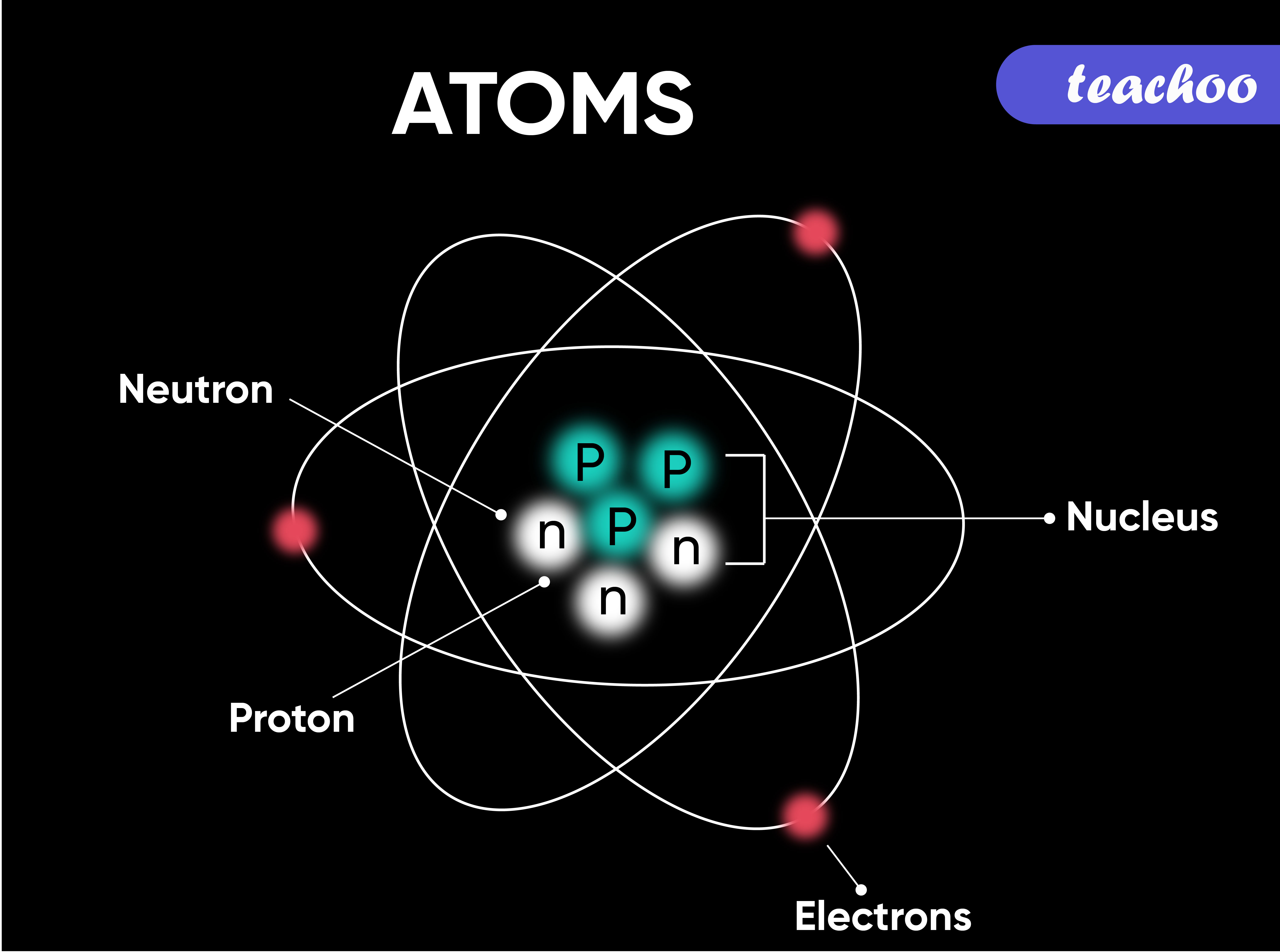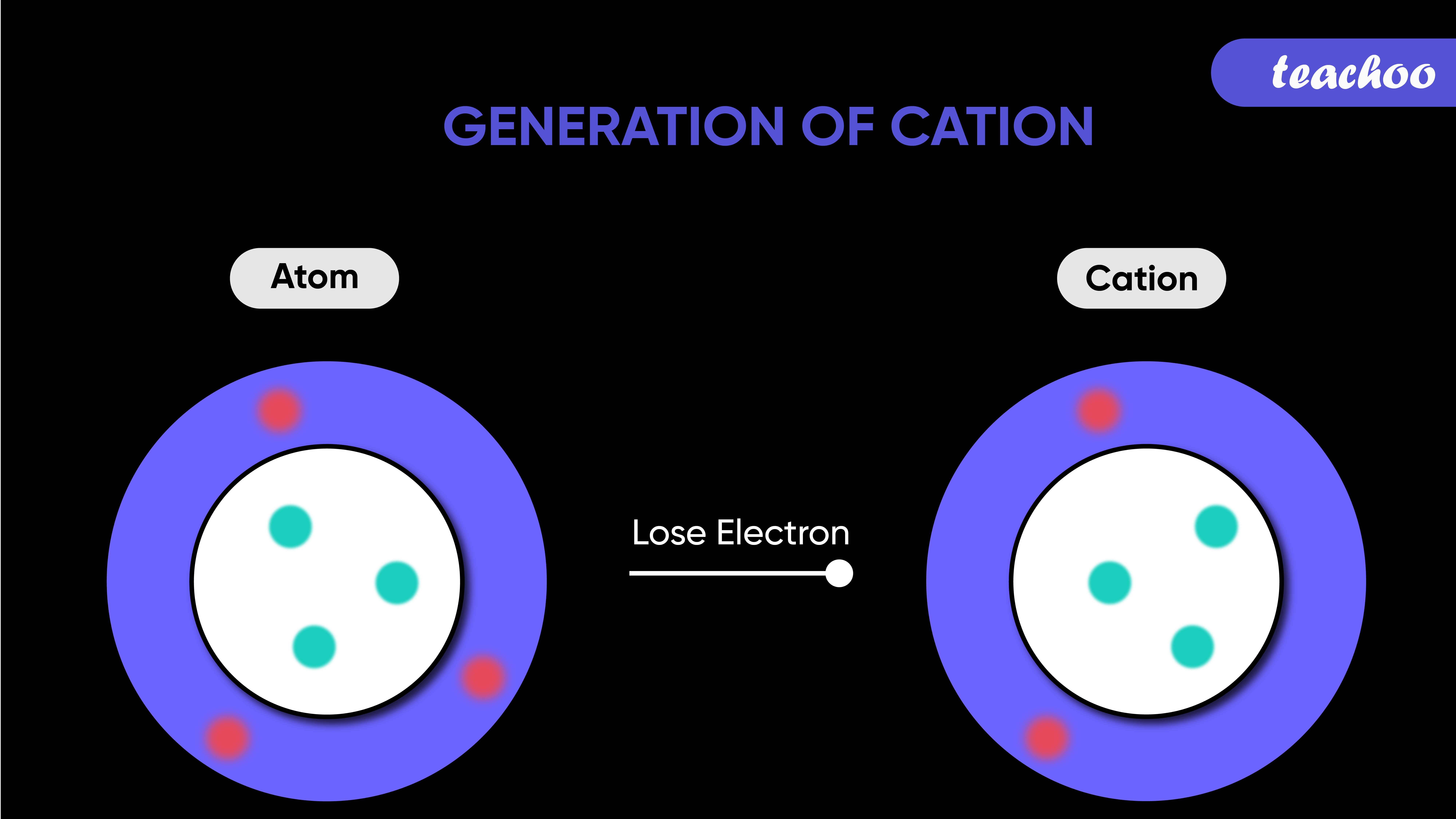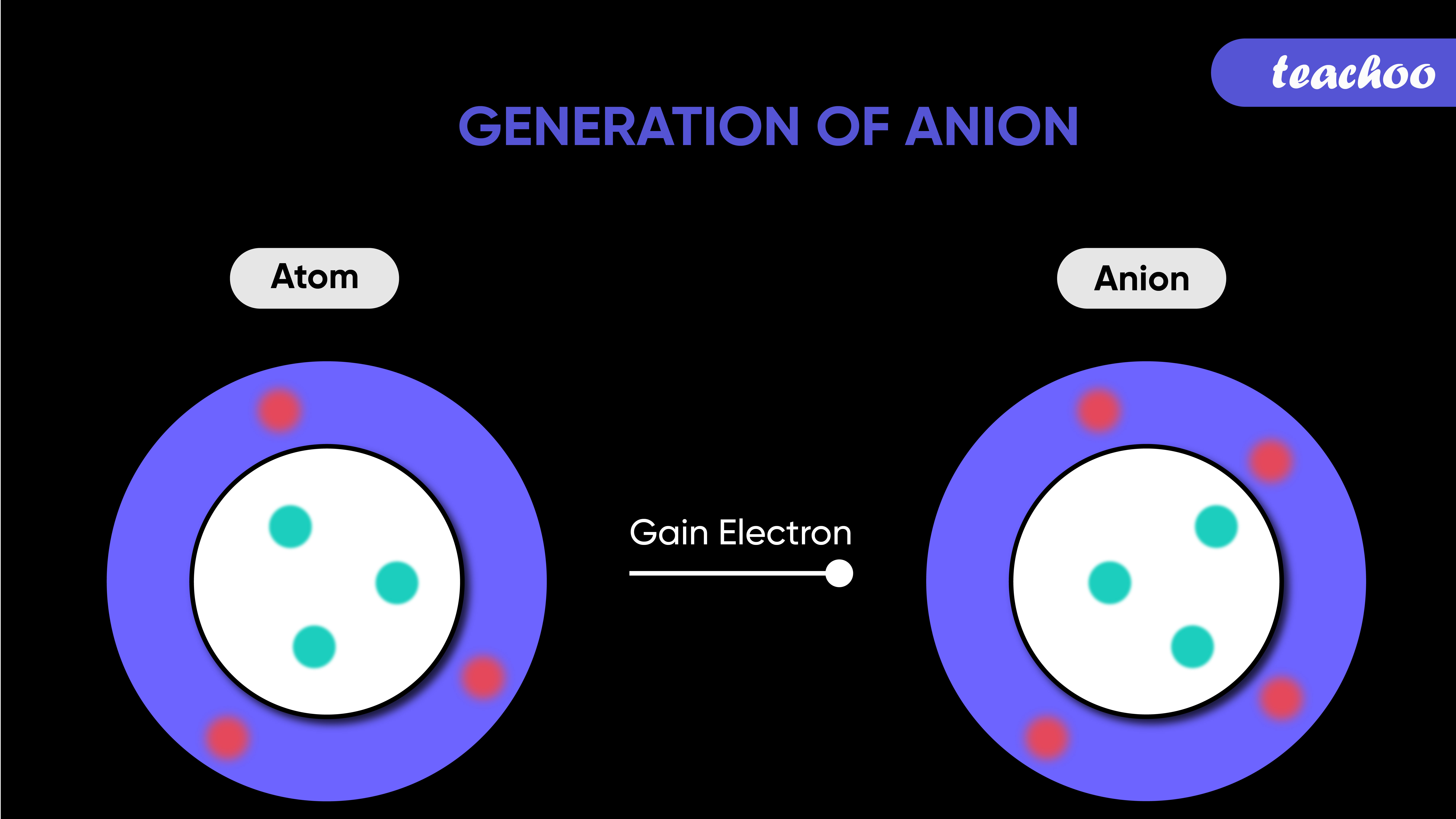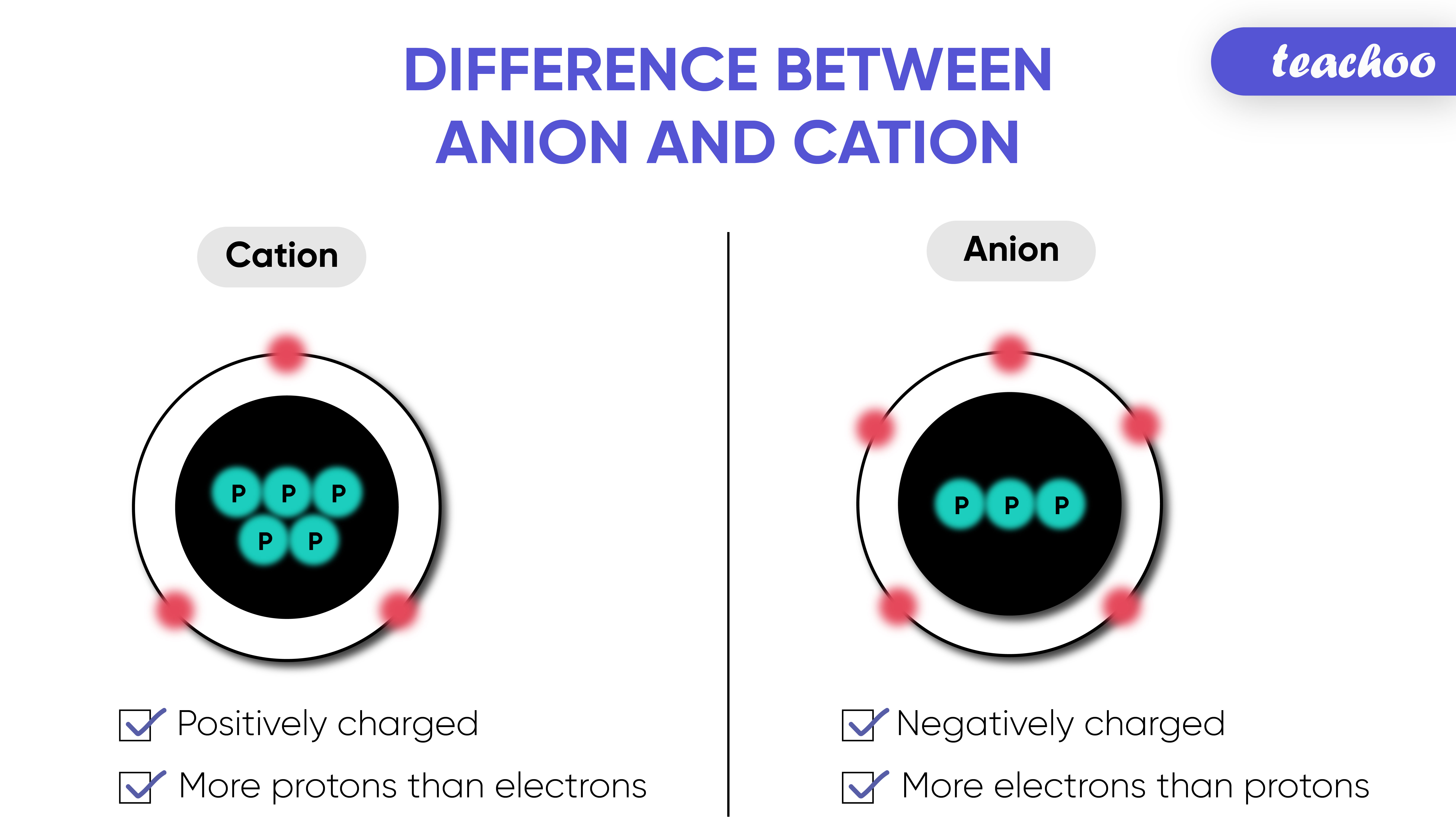## What is Ion?

A charged atom or a group of charged atoms is called ions

It may be positively or negatively charged

Positively charged ion is called Cation

Negatively charged ion is called Anion

A group of atoms carrying a charge is known as a polyatomic ion.
For example - NH 4 +,NO 3 -

## What is a Molecule?

A group of atoms chemically bonded together is Here are the valency of a few elements:

called Molecule.

## How is Ion formed?

We know that atom contains protons, neutrons and electrons

• Protons are Positively charged
• Electrons are Negatively charged
• Neutrons are NeutralIn an atom, the number of protons is equal to the number of electrons.
So the positive and negative charges are balanced out

Ions are formed when atoms lose or gain electrons

## What is a Molecule?

A group of atoms chemically bonded together is Here are the valency of a few elements:
called Molecule.

So,

## Case 1: When an atom loses an electron,

The negative charge reduces.
Thus it becomes positively charged - Cation, because;
The number of positively charged protons becomes more than negatively charged electrons## What is a Molecule?

A group of atoms chemically bonded together is Here are the valency of a few elements:
called Molecule.

## Case 2: When atom gains an electron,

The negative charge increases.
Thus it becomes negatively charged - Anion, because;
The number of negatively charged electrons becomes more than positively charged protons## What is a Molecule?

A group of atoms chemically bonded together is Here are the valency of a few elements:
called Molecule.

## Difference between Anion and Cation

 Cation Anion Positively charged ion is called Cation Negatively charged ion is called anion It is formed when atom loses electron (and hence become positively charged) It is formed when atom gains electron and become positively charged Example - Sodium Ion (Na + ) Example - Chloride Ion (Cl - ) Sodium (Na) has 11 protons and 11 electrons Overall charge is 0 Chlorine (Cl) has 17 protons and 17 electrons Overall charge is 0 When sodium loses 1 electron It has 11 protons and 10 electrons Positive charge > Negative charge Thus becomes Cation (Na + ) When chlorine gains 1 electrons It has 17 protons and 18 electrons Negative charge > Positive charge Thus becomes Anion (Cl - )## What is a Molecule?

A group of atoms chemically bonded together is Here are the valency of a few elements:

called Molecule.

Example -
In text Question -
NCERT Question -

1. Class 9
2. Chapter 3 Class 9 - Atoms And Molecules (Term 2)
3. Concepts

Concepts

Class 9
Chapter 3 Class 9 - Atoms And Molecules (Term 2)